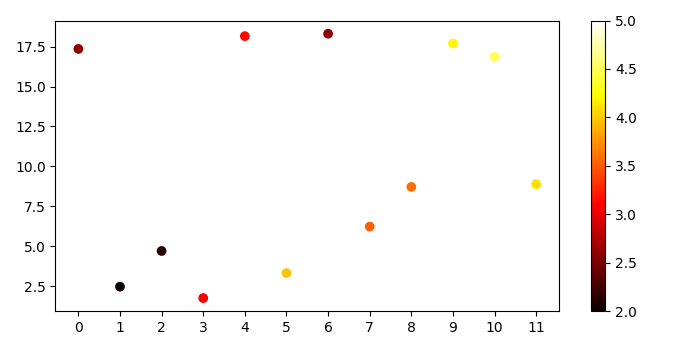# How can I convert numbers to a color scale in Matplotlib?

To convert numbers to a color scale in matplotlib, we can take the following steps.

## Steps

• Create x, y and c data points using numpy.
• Convert the data points to Pandas dataframe.
• Create a new figure or activate an existing figure using subplots() method.
• Get the hot colormap.
• To linearly normalize the data, we can use Normalize() class.
• Plot the scatter points with x and y data points and linearly normalized colormap.
• Set the xticks for x data points.
• To make the colorbar, create a scalar mappable object.
• Use colorbar() method to make the colorbar.
• To display the figure, use show() method.

## Example

from matplotlib import pyplot as plt, colors
import numpy as np
import pandas as pd
plt.rcParams["figure.figsize"] = [7.00, 3.50]
plt.rcParams["figure.autolayout"] = True
x = np.arange(12)
y = np.random.rand(len(x)) * 20
c = np.random.rand(len(x)) * 3 + 1.5
df = pd.DataFrame({"x": x, "y": y, "c": c})
fig, ax = plt.subplots()
cmap = plt.cm.hot
norm = colors.Normalize(vmin=2.0, vmax=5.0)
ax.scatter(df.x, df.y, color=cmap(norm(df.c.values)))
ax.set_xticks(df.x)
sm = plt.cm.ScalarMappable(cmap=cmap, norm=norm)
fig.colorbar(sm)
plt.show()

## Output